# Power Analyses - Workshop

#### 22/2/2021

library(lmerTest)
library(afex)
library(ggplot2)
library(dplyr)
library(designr)
library(gridExtra)
library(MASS)

# 1 What is statistical power?

• Given the H1 is true
• Given we assume some effect size
• –> Power = probability to obtain a significant result; i.e., to correctly recover the true H1

We can compute power by

• assuming the H1 is true
• assuming some effect size (e.g., difference in DV between 2 conditions)

How to obtain the probability of a significant result?

• simulate data based on the assumed model + effect size
• do this many times (e.g., nsim = 1000)
• run the statistical model on each simulated data set
• determine how many times we find a significant effect –> this probability is = power

## 1.1 An easy example: 2-sample t-test

# a) assume effect size + simulate data
x <- rep(c("x1","x2"), each=30)
y <- rep(c( 200, 220), each=30) + rnorm(60,0,50)
ggplot(data.frame(x,y), aes(x=x,y=y)) + geom_boxplot()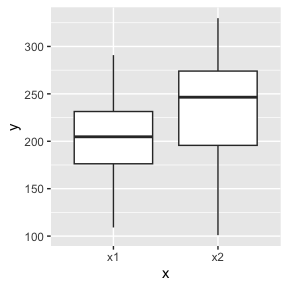# b) run statistical model & get p-value
t.test(y ~ x)$p.value ##  0.03388535 # c) do this many times nsim <- 1000 pVal <- c() for (i in 1:nsim) { x <- rep(c("x1","x2"), each=30) y <- rep(c( 200, 220), each=30) + rnorm(60,0,50) # b) run statistical model & get p-value pVal[i] <- t.test(y ~ x)$p.value
}
# d) check how often it is significant
(power <- mean( pVal < 0.05 ))
##  0.337
# e)
# We could do this for different numbers of subjects and see when power is ~80%
# We could change the difference in means/residual standard deviations

What we usually want to achieve is a power of 80%. That’s the standard. We should plan our analysis, with the number of subjects and items to achieve this power value of 80%. That is, if the effect that we want to test really exists, we want to have an 80% chance of actually detecting the effect with our analysis. In the power analysis, we can then vary the number of subjects or items, or make different assumptions about the effect size to see what we need to achieve 80% power. This should be the basis for planing an experimental study. Note that in the simulation studies below, we will sometimes use power thresholds of lower than 80%. This should normally not be done when running empirical studies.

One more point about power: if an experimental design has low power, then this can have severe negative consequences for statistical analysis. In an extreme case where power is only 10%, then if we find a significant result, there is a very high chance that the effect is actually due to chance and that the H0 is true. Moreover, when we have low power, then the effect size estimates from significant results are guaranteed to be too large (type M = magnitude error), and there is a danger finding effects in the wrong direction (type S = sign error).

Therefore, it is important to plan studies such that they have a good power, ideally = 80%. If you want to provide evidence for the null hypothesis, then even a higher power of e.g., 95% can be beneficial.

### 1.1.1 Linear model formulation

This is the formula for a simple linear model: $$y_i = b_0 + b_1 * x_i + e_i$$, where $$y_i$$ is the dependent variable for subject $$i$$, $$b_0$$ is the intercept, $$b_1$$ is the slope, $$x_i$$ is the value of the predictor for subject $$i$$, and $$e_i$$ is the random error term for subject $$i$$. We can use this to simulate data.

The predictor term: This could be a continuous variable in a linear regression analysis (e.g., $$x_i$$ could indicate the age of subject $$i$$). However, we can also use this formulation if the predictor is a factor, e.g., if we have two groups of subjects “x1” and “x2”. In this case, we have to use contrasts to code factors into numbers (for contrasts see Schad et al., 2020, JML). One example is the sum contrast. Here, the predictor variable is $$-1$$ for one group (e.g., for x1) and $$+1$$ for the other group (e.g., for x2).

# previous formulation
x <- rep(c("x1","x2"), each=30)
y <- rep(c( 200, 220), each=30) + rnorm(60,0,50)
# new:
x <- rep(c(-1,+1), each=30) # define predictor term: here, sum contrast coding (-1, +1)
y <- 210 + 10*x + rnorm(60,0,50) # simulate data
# run linear model
lm(y ~ x)
##
## Call:
## lm(formula = y ~ x)
##
## Coefficients:
## (Intercept)            x
##     207.735       -1.415
summary(lm(y ~ x))
##
## Call:
## lm(formula = y ~ x)
##
## Residuals:
##     Min      1Q  Median      3Q     Max
## -98.808 -30.032   3.904  37.831  70.513
##
## Coefficients:
##             Estimate Std. Error t value Pr(>|t|)
## (Intercept)  207.735      5.649   36.77   <2e-16 ***
## x             -1.415      5.649   -0.25    0.803
## ---
## Signif. codes:  0 '***' 0.001 '**' 0.01 '*' 0.05 '.' 0.1 ' ' 1
##
## Residual standard error: 43.76 on 58 degrees of freedom
## Multiple R-squared:  0.00108,    Adjusted R-squared:  -0.01614
## F-statistic: 0.06273 on 1 and 58 DF,  p-value: 0.8031
ggplot(data.frame(x,y), aes(x=factor(x),y=y)) + geom_boxplot()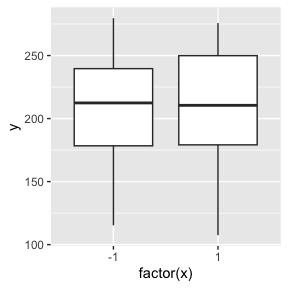# 2 Content

1. ANOVA + LMM: Random effects for subjects only, 1 within-subject factor
2. ANOVA + LMM: Random effects for subjects only, 2x3 within-subject design
3. LMM: Crossed random effects for subjects and items
4. Vary effect size
5. Based on a previous data set: latin square design
6. LMM: continuous covariate
7. logistic GLMM

# 3 Steps of a power analysis

1. Create experimental design (designr)
1. Simulate data (simLMM)
1. Run statistical model (lmer, aov_car)
1. Do this many times and compute power

Other statistical software: R-packages simr and faux

## 3.1 a) Create experimental design (desinr)

The R-package designr has been written by us to create factorial experimental designs.

library(designr)

# one within-subject factor
# create design
design <-
fixed.factor("X", levels=c("X1", "X2")) + # fixed effect
random.factor("Subj", instances=5)        # random effect
dat <- design.codes(design) # create data frame (tibble) from experimental design
length(unique(dat$Subj)) # number of subjets ##  5 data.frame(dat) # look at data ## Subj X ## 1 Subj1 X1 ## 2 Subj1 X2 ## 3 Subj2 X1 ## 4 Subj2 X2 ## 5 Subj3 X1 ## 6 Subj3 X2 ## 7 Subj4 X1 ## 8 Subj4 X2 ## 9 Subj5 X1 ## 10 Subj5 X2 # one between-subject factor design <- fixed.factor("X", levels=c("X1", "X2")) + random.factor("Subj", groups="X", instances=5) dat <- design.codes(design) length(unique(dat$Subj))
##  10
data.frame(dat)
##      Subj  X
## 1  Subj01 X1
## 2  Subj02 X1
## 3  Subj03 X1
## 4  Subj04 X1
## 5  Subj05 X1
## 6  Subj06 X2
## 7  Subj07 X2
## 8  Subj08 X2
## 9  Subj09 X2
## 10 Subj10 X2
# 2 (A: within-subjects) x 2 (B: between-subjects) design
design <-
fixed.factor("A", levels=c("A1", "A2")) +
fixed.factor("B", levels=c("B1", "B2")) +
random.factor("Subj", groups="B", instances=5)
dat <- design.codes(design)
length(unique(dat$Subj)) ##  10 data.frame(dat) ## Subj A B ## 1 Subj01 A1 B1 ## 2 Subj01 A2 B1 ## 3 Subj02 A1 B1 ## 4 Subj02 A2 B1 ## 5 Subj03 A1 B1 ## 6 Subj03 A2 B1 ## 7 Subj04 A1 B1 ## 8 Subj04 A2 B1 ## 9 Subj05 A1 B1 ## 10 Subj05 A2 B1 ## 11 Subj06 A1 B2 ## 12 Subj06 A2 B2 ## 13 Subj07 A1 B2 ## 14 Subj07 A2 B2 ## 15 Subj08 A1 B2 ## 16 Subj08 A2 B2 ## 17 Subj09 A1 B2 ## 18 Subj09 A2 B2 ## 19 Subj10 A1 B2 ## 20 Subj10 A2 B2 # 1 factor, 2 (crossed) random effects, within-subject, between-item factor design <- fixed.factor("X", levels=c("X1", "X2")) + random.factor("Subj", instances=5) + random.factor("Item", groups="X", instances=2) dat <- design.codes(design) length(unique(dat$Subj))
##  5
length(unique(dat$Item)) ##  4 data.frame(dat) ## Subj Item X ## 1 Subj1 Item1 X1 ## 2 Subj1 Item2 X1 ## 3 Subj1 Item3 X2 ## 4 Subj1 Item4 X2 ## 5 Subj2 Item1 X1 ## 6 Subj2 Item2 X1 ## 7 Subj2 Item3 X2 ## 8 Subj2 Item4 X2 ## 9 Subj3 Item1 X1 ## 10 Subj3 Item2 X1 ## 11 Subj3 Item3 X2 ## 12 Subj3 Item4 X2 ## 13 Subj4 Item1 X1 ## 14 Subj4 Item2 X1 ## 15 Subj4 Item3 X2 ## 16 Subj4 Item4 X2 ## 17 Subj5 Item1 X1 ## 18 Subj5 Item2 X1 ## 19 Subj5 Item3 X2 ## 20 Subj5 Item4 X2 # within-subject, within-item factor design <- fixed.factor("X", levels=c("X1", "X2")) + random.factor("Subj", instances=5) + random.factor("Item", instances=2) dat <- design.codes(design) length(unique(dat$Subj))
##  5
length(unique(dat$Item)) ##  2 data.frame(dat) ## Subj Item X ## 1 Subj1 Item1 X1 ## 2 Subj1 Item1 X2 ## 3 Subj1 Item2 X1 ## 4 Subj1 Item2 X2 ## 5 Subj2 Item1 X1 ## 6 Subj2 Item1 X2 ## 7 Subj2 Item2 X1 ## 8 Subj2 Item2 X2 ## 9 Subj3 Item1 X1 ## 10 Subj3 Item1 X2 ## 11 Subj3 Item2 X1 ## 12 Subj3 Item2 X2 ## 13 Subj4 Item1 X1 ## 14 Subj4 Item1 X2 ## 15 Subj4 Item2 X1 ## 16 Subj4 Item2 X2 ## 17 Subj5 Item1 X1 ## 18 Subj5 Item1 X2 ## 19 Subj5 Item2 X1 ## 20 Subj5 Item2 X2 # within-subject, within-item factor, latin square design design <- fixed.factor("X", levels=c("X1", "X2")) + random.factor("Subj", instances=5) + random.factor("Item", instances=2) + random.factor(c("Subj", "Item"), groups=c("X")) dat <- design.codes(design) length(unique(dat$Subj))
##  10
length(unique(dat$Item)) ##  4 data.frame(dat) ## Subj Item X ## 1 Subj01 Item1 X1 ## 2 Subj01 Item2 X2 ## 3 Subj01 Item3 X1 ## 4 Subj01 Item4 X2 ## 5 Subj02 Item1 X2 ## 6 Subj02 Item2 X1 ## 7 Subj02 Item3 X2 ## 8 Subj02 Item4 X1 ## 9 Subj03 Item1 X1 ## 10 Subj03 Item2 X2 ## 11 Subj03 Item3 X1 ## 12 Subj03 Item4 X2 ## 13 Subj04 Item1 X2 ## 14 Subj04 Item2 X1 ## 15 Subj04 Item3 X2 ## 16 Subj04 Item4 X1 ## 17 Subj05 Item1 X1 ## 18 Subj05 Item2 X2 ## 19 Subj05 Item3 X1 ## 20 Subj05 Item4 X2 ## 21 Subj06 Item1 X2 ## 22 Subj06 Item2 X1 ## 23 Subj06 Item3 X2 ## 24 Subj06 Item4 X1 ## 25 Subj07 Item1 X1 ## 26 Subj07 Item2 X2 ## 27 Subj07 Item3 X1 ## 28 Subj07 Item4 X2 ## 29 Subj08 Item1 X2 ## 30 Subj08 Item2 X1 ## 31 Subj08 Item3 X2 ## 32 Subj08 Item4 X1 ## 33 Subj09 Item1 X1 ## 34 Subj09 Item2 X2 ## 35 Subj09 Item3 X1 ## 36 Subj09 Item4 X2 ## 37 Subj10 Item1 X2 ## 38 Subj10 Item2 X1 ## 39 Subj10 Item3 X2 ## 40 Subj10 Item4 X1 # 4 ANOVA + LMM: Random effects for subjects only, 1 within-subject factor Ok, let’s run a power analysis for a simple example: one within-subject factor with subject random effects. design <- fixed.factor("X", levels=c("X1", "X2")) + random.factor("Subj", instances=30) dat <- design.codes(design) length(unique(dat$Subj))
##  30
(contrasts(dat$X) <- c(-1, +1)) # define a sum contrast ##  -1 1 dat$Xn <- model.matrix(~X, dat)[,2] # convert this it a numeric variable

Next, we will use the R-function simLMM() to simulate data for a linear mixed-effects model. simLMM() is currently under development. If you find any errors, please write them into a script that reproduces the error and send it to us (e.g., to ). This would be very important feedback! Thank you for your help.

How does the function work? The most important function arguments are the following:

simLMM(formula, data, Fixef, VC_sd, CP)

Formula is a standard formula used in the lmer function. For the present example, the formula would be form = ~ 1 + X + (1 + X | Subj). Since simLMM is a simulation function, we do not have to specify a dependent variable. This example indicates an intercept 1 and a fixed effect for factor X. For the random effects, we see random effects for subjects Subj; these specify a random intercept and random slopes for factor X. Since there is only one pipe |, we know that the random intercepts and slopes are assumed to be correlated.

The next argument is the data data, which is a data frame containing all the variables - this is just the same as in the lmer function. Here, our data frame was termed dat, i.e., data=dat.

The next argument Fixef specifies the fixed effects. This is a vector of numbers that specify the regression coefficients / fixed effects for each term in the formula. In our example, we have two fixed effects, an intercept and a slope. Thus, we enter two numbers, e.g., Fixef = c(200, 10), which specifies an intercept of $$200$$ and a slope of $$10$$.

The next argument VC_sd specifies the standard deviations for the random effects. It is entered as a list. In the present example, we have only two random effects: subjects and the residual noise. These are assumed to be normally distributed with mean zero and some standard deviation. For subjects we have two random effects terms, the intercept and the random slopes. Let’s assume the intercept varies across subjects with a standard deviation of $$30$$ and the slope varies across subjects with a standard deviation of $$10$$. We combine these two terms in one vector sd_Subj <- c(30, 10). The second random effects term is the residual standard deviation. We here assume the residual noise is normally distributed with a mean of $$0$$ and some standard deviation, which we assume here is $$50$$. We now combine these standard deviations into a list VC_sd = list( c(30, 10), 50): we first enter the standard deviations for the subject random effects as a vector, and then we enter the standard deviation for the residual noise.

The next argument CP specifies the random effects correlations. Here, we have different options for how to specify this. One option is to simply specify a single number, e.g., CP=0.3. This will set all random effects correlations in the model to the value $$0.3$$. If we have several random effects terms, e.g., for subjects and for items, we can enter a vector of length two, where the first number would specify all random effects correlations for subjects, and the second would specify all random effects correlations for items. Last, we can also enter a list of correlation matrices. E.g., when we have subjects and items, we would have a list of length two. And the first entry would contain a correlation matrix for the subject random effects and the second entry would contain a correlation matrix for item random effects. Here, because we have only one random effects correlation in the model, we simply use the specification CP=0.3.

Taken together, this gives the following call of the simLMM function: simLMM(~ X + (X | Subj), data=dat, Fixef=fix, VC_sd=list(sd_Subj, sd_Res), CP=0.3).

One additional argument that we can use is empirical. By default this is set to empirical=FALSE. If we set this to empirical=TRUE, then the fixed effects estimates in the simulated data will exactly correspond to the numbers that we specify. However, the random effects will not be exact. We will demonstrate this below. If we use the default empirical=FALSE, then the numbers (fixed-effects + random effects) that we specify are the “true” population parameters, from which we draw a random sample in the simulation. Note that empirical=TRUE does not work for data from a logistic GLMM and it does not work when you have continuous predictors, i.e., covariates, instead of/in addition to factors.

With this background, let’s simulate data for our within-subject design. We use empirical=TRUE.

# simulate data
fix     <- c(200,10) # set fixed effects
sd_Subj <- c(30, 10) # set random effects standard deviations for subjects
sd_Res  <- 50        # set residual standard deviation
dat$ysim <- simLMM(form = ~ 1 + X + (1 + X | Subj), data = dat, Fixef = fix, VC_sd = list(sd_Subj, sd_Res), CP = 0.3, empirical = TRUE) ## Data simulation from a linear mixed-effects model (LMM) ## Formula: gaussian ~ 1 + X1 + ( 1 + X1 | Subj ) ## empirical = TRUE ## Random effects: ## Groups Name Std.Dev. Corr ## Subj (Intercept) 30.0 ## X1 10.0 0.30 ## Residual 50.0 ## Number of obs: 60, groups: Subj, 30 ## Fixed effects: ## (Intercept) X1 ## 200 10 We see that the simLMM function produces some output that looks very similar to the output from a fitted lmer object. In this output, we can check whether our specification of fixed and random effects is what we intended, or whether we made some mistake in our specification. We can turn this output off by specifying verbose=FALSE. This will be useful in power simulations where we simulate data many many times. Next, let’s check the simulated data. # check group means dat %>% group_by(X) %>% summarize(M=mean(ysim)) # the group means are EXACTLY 190 and 210. This is due to empirical=TRUE. ## # A tibble: 2 × 2 ## X M ## <fct> <dbl> ## 1 X1 190 ## 2 X2 210 # check fixed effects + random effects (m1 <- lmer(ysim ~ Xn + (Xn || Subj), data=dat, control=lmerControl(calc.derivs=FALSE))) # The fixed effects are EXACTLY as indicated. This is due to empirical=TRUE. ## Warning in as_lmerModLT(model, devfun): Model may not have converged with 1 ## eigenvalue close to zero: 1.7e-09 ## Linear mixed model fit by REML ['lmerModLmerTest'] ## Formula: ysim ~ Xn + (Xn || Subj) ## Data: dat ## REML criterion at convergence: 646.0295 ## Random effects: ## Groups Name Std.Dev. ## Subj (Intercept) 38.20 ## Subj.1 Xn 28.89 ## Residual 35.46 ## Number of obs: 60, groups: Subj, 30 ## Fixed Effects: ## (Intercept) Xn ## 200 10 aov_car(ysim ~ 1 + Error(Subj/X), data=dat) # We can also run an ANOVA ## Anova Table (Type 3 tests) ## ## Response: ysim ## Effect df MSE F ges p.value ## 1 X 1, 29 2926.46 2.05 .028 .163 ## --- ## Signif. codes: 0 '***' 0.001 '**' 0.01 '*' 0.05 '+' 0.1 ' ' 1 We can see that because the setting empirical=TRUE, the fixed effects were estimated exactly to the numbers that we set in the specification. This suggests that the simulation process was implemented as intended. However, the random effects terms are not exact. VERY IMPORTANT: In order to perform a power analysis, we have to use empirical=FALSE. In a power analysis, we are assuming that we randomly sample from the population parameters. This is what would happen in a “real” experiment. And we want to know how often an effect is significant. Next, we perform a power analysis. We want to find out how many subjects are needed to run this study. We assume the fixed and random effects specified above. We simulate data from the model for different numbers of subjects. And we then fit lmer models to the simulated data to see how often the effect of factor X is significant given that there is a true effect of X in the data. nsubj <- seq(10,100,1) # we vary the number of subjects from 10 to 100 in steps of 1 nsim <- length(nsubj) # number of simulations COF <- COFaov <- data.frame() warn <- c() for (i in 1:nsim) { # i <- 1 #print(paste0(i,"/",nsim)) # create experimental design design <- fixed.factor("X", levels=c("X1", "X2")) + random.factor("Subj", instances=nsubj[i]) dat <- design.codes(design) nsj <- length(unique(dat$Subj))
# create contrast and store as numeric variable
contrasts(dat$X) <- c(-1, +1) dat$Xn <- model.matrix(~X,dat)[,2]
# for each number of subjects, we run 10 simulations to obtain more stable results
for (j in 1:10) { # j <- 1
# simulate data
dat$ysim <- simLMM(~ X + (X | Subj), data=dat, Fixef=fix, VC_sd=list(sd_Subj, sd_Res), CP=0.3, empirical=FALSE, verbose=FALSE) # ANOVA AOV <- aov_car(ysim ~ 1 + Error(Subj/X), data=dat) AOVcof <- summary(AOV)$univariate.tests[2,]
COFaov <- rbind(COFaov,c(nsj,AOVcof))
# LMM
#LMM <- lmer(ysim ~ Xn + (Xn || Subj), data=dat,
#            REML=FALSE, control=lmerControl(calc.derivs=FALSE))
# run lmer and capture any warnings
ww <- ""
suppressMessages(suppressWarnings(
LMM <- withCallingHandlers({
lmer(ysim ~ Xn + (Xn || Subj), data=dat, REML=FALSE,
control=lmerControl(calc.derivs=FALSE))
},
warning = function(w) { ww <<- w$message } ) )) LMMcof <- coef(summary(LMM))[2,] COF <- rbind(COF,c(nsj,LMMcof,isSingular(LMM))) warn[i] <- ww } } #load(file="data/Power0.rda") # Results for LMMs names(COF) <- c("nsj","Estimate","SE","df","t","p","singular") COF$warning <- warn
COF$noWarning <- warn=="" COF$sign   <- as.numeric(COF$p < .05 & COF$Estimate>0) # determine significant results
COF$nsjF <- gtools::quantcut(COF$nsj, q=seq(0,1,length=10))
COF$nsjFL <- plyr::ddply(COF,"nsjF",transform,nsjFL=mean(nsj))$nsjFL
# Results for ANOVAs
COFaov$sign <- as.numeric(COFaov$p < .05) # determine significant results
COFaov$nsjF <- gtools::quantcut(COFaov$nsj, q=seq(0,1,length=10))
COFaov$nsjFL <- plyr::ddply(COFaov,"nsjF",transform,nsjFL=mean(nsj))$nsjFL
#save(COF, COFaov, file="data/Power0.rda")

Note that in a standard LMM analysis, we would be interested to fit a parsimonious LMM (Matuschek et al., 2017, JML). This involves careful selection of the random effects. Unless one has an automatized selection of the parsimonious LMM, this is not possible when performing a power analysis and fitting hundreds of models. An alternative possibility is to fit a model with all random slopes, but with no random effects correlations. The problem with random effects correlation is that they are often very hard to estimate from the data. When trying to estimate them, there are often failures in model fit. However, removing the random effects correlations from the fit presumably does not affect power and alpha error much (Barr et al., 2013, JML). Therefore, for power analyses, it is recommended to not estimate random effects correlations. However, normally all random slopes should be estimated, since neglecting random slopes in the estimation can sometimes heavily inflate the alpha error and lead to false positive results (i.e., if there is strong true variation in the effect; Barr et al., 2013, JML).

First, we should check whether the models were singular fits. Singular fits are cases where some of the parameter values were estimated on their boundary. For example, a random effects standard deviation can be estimated to be exactly $$0$$, or a correlation parameter can be estimated to be exactly $$+1$$ or $$-1$$. Normally, this should be avoided in LMMs, usually by excluding the respective term from the model. However, this is not possible in power analyses due to the large number of model fits. Therefore, we here check in how many cases there are singular fits.

load(file=system.file("power-analyses", "Power0.rda", package = "designr"))

mean(COF$singular) ##  0 The mean of zero indicates that none of the models were singular fits. This supports the results from the analysis. Moreover, we can check whether there were any warning messages, i.e., whether the optimizer has converged for the models. mean(COF$noWarning)
##  0.4945055

This shows that 50% of fits showed no warning message, which also means that 50% of model fits did exhibit a warning message. This is very bad news. It probably means that we cannot use this power analysis. Indeed, if we would run a study, there would be a high chance that there is a problem with convergence.

COF$warning[1:20] ##  "" ##  "Model may not have converged with 1 eigenvalue close to zero: 6.7e-10" ##  "" ##  "" ##  "Model failed to converge with 1 negative eigenvalue: -1.5e-06" ##  "" ##  "" ##  "Model may not have converged with 1 eigenvalue close to zero: -1.3e-09" ##  "Model failed to converge with 1 negative eigenvalue: -4.2e-07" ##  "" ##  "" ##  "" ##  "Model failed to converge with 1 negative eigenvalue: -6.0e-08" ##  "" ##  "Model may not have converged with 1 eigenvalue close to zero: -6.3e-09" ##  "" ##  "Model failed to converge with 1 negative eigenvalue: -1.8e-07" ##  "" ##  "Model failed to converge with 1 negative eigenvalue: -1.8e-07" ##  "" Notice that we haven’t yet implemented the detection of warning messages for the other example analyses (see below). But this should be done when conducting a power analysis. There is a paper that argues that warning messages can be largely ignored (https://debruine.github.io/lmem_sim/articles/paper.html): “as long as there are not too many of these non-convergence warnings relative to the number of runs, you can probably ignore them because the won’t affect the overall estimates”. However, with 50% warnings, we definitely have a problem. One possible option could be to see whether the average effect estimate (e.g. for the fixed effects) is the same for fits with versus without convergence warning. One reason why we have many warnings here is because we used a small number of data points to speed up the fitting algorithm. Advice: (This advice is off the top of the head; not based on evidence.) 1. If there are more than 1% singular fits, warnings about eigenvalues, model crashes, etc. then don’t use this design and respecify the model with more subjects and / or items or simplify the random-effect structure (e.g., remove VCs which you expect to be small). If a simulation passes (1), 1. Ignore the <=1% problem cases 2. Determine power for critical effects, VCs, and CPs — assuming that you want to determine the number of Subj and number of Item for 80% power for the effect sizes you expect. Because this is an artificial example, we now proceed to plot the percentage of significant results as a function of the number of subjects. p1 <- ggplot(data=COF)+ geom_smooth(aes(x=nsj, y=sign))+ geom_point( stat="summary", aes(x=nsjFL, y=sign))+ geom_errorbar(stat="summary", aes(x=nsjFL, y=sign))+ geom_line( stat="summary", aes(x=nsjFL, y=sign)) p2 <- ggplot(data=COFaov)+ geom_smooth(aes(x=nsj, y=sign))+ geom_point( stat="summary", aes(x=nsjFL, y=sign))+ geom_errorbar(stat="summary", aes(x=nsjFL, y=sign))+ geom_line( stat="summary", aes(x=nsjFL, y=sign)) grid.arrange(p1,p2, nrow=1)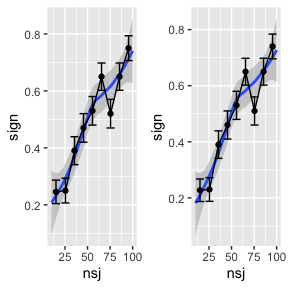We can also run a local regression analysis (i.e., the smoothed regression line) manually and determine the number of subjects that we need to obtain a power of 60%. # determine number of subjects needed for a power of 60% m0 <- loess(sign ~ nsj, data=COF) COF$pred <- predict(m0)
idx <- COF$pred>0.6 min(COF$nsj[idx])
##  71

# 5 ANOVA + LMM: Random effects for subjects only, 2x3 within-subject design

We now create a 2 x 3 within-subject design with random effects for subjects.

design <-
fixed.factor("A", levels=c("A1", "A2")) +
fixed.factor("B", levels=c("B1", "B2", "B3")) +
random.factor("Subj", instances=60) #+
#random.factor("Items", instances=5)
dat <- design.codes(design)
nrow(dat)
##  360
length(unique(dat$Subj)) ##  60 head(dat,12) ## # A tibble: 12 × 3 ## Subj A B ## <fct> <fct> <fct> ## 1 Subj01 A1 B1 ## 2 Subj01 A2 B1 ## 3 Subj01 A1 B2 ## 4 Subj01 A2 B2 ## 5 Subj01 A1 B3 ## 6 Subj01 A2 B3 ## 7 Subj02 A1 B1 ## 8 Subj02 A2 B1 ## 9 Subj02 A1 B2 ## 10 Subj02 A2 B2 ## 11 Subj02 A1 B3 ## 12 Subj02 A2 B3 # set contrasts (contrasts(dat$A) <- c(-1, +1))
##  -1  1
(contrasts(dat$B) <- contr.sdif(3)) ## 2-1 3-2 ## 1 -0.6666667 -0.3333333 ## 2 0.3333333 -0.3333333 ## 3 0.3333333 0.6666667 # Recommendation: hypr-package for contrasts + Schad et al. (2020) JML dat$An  <- model.matrix(~A,dat)[,2]
dat[,c("Bn1","Bn2")] <- model.matrix(~B,dat)[,2:3]

Note that we use a sliding difference contrast (contr.sdif) for the 3-level factor B. The first of these contrasts tests the difference between B2 and B1, the second contrast tests the difference between B3 and B2.

One option would now be to specify the relevant fixed and random effects that relate to the set contrasts. This would imply defining a fixed effect for the intercept, one for the effect of factor A, two for the effect of factor B (which is coded via two contrasts), and two effects for the interaction of A x B. The fixed-effects part of the formula would look like this: ~ An + Bn1 + Bn2 + An:Bn1 + An:Bn2, and we could specify fixed effects as Fixef=c(200,0,0,0,-1,-1). A similar specification would be needed for the random effects part. This would be a good approach.

One issue here might be that we might find it difficult to specify our expectations in terms of these fixed effects. Maybe we find it easier to specify the expected group means. We can use a trick to specify the group means instead of the fixed effects written down above. We create one large factor that has each design cell as a separate factor level: dat$F <- factor(paste0(dat$A, ".", dat$B)). Now, in the specification of the model formula, we can remove the intercept term (i.e., via -1 or 0 +). If we would do so in a call to lmer (or equivalently to lm), then the fixed effects would simply provide estimates of the condition means, i.e., the mean responses for each factor level. We can take a look at how this factor is coded in the design matrix by using the function model.matrix(). # create one factor dat$F <- factor(paste0(dat$A, ".", dat$B))
levels(dat$F) ##  "A1.B1" "A1.B2" "A1.B3" "A2.B1" "A2.B2" "A2.B3" mm <- model.matrix(~ -1 + F, data=dat) head(mm) ## FA1.B1 FA1.B2 FA1.B3 FA2.B1 FA2.B2 FA2.B3 ## 1 1 0 0 0 0 0 ## 2 0 0 0 1 0 0 ## 3 0 1 0 0 0 0 ## 4 0 0 0 0 1 0 ## 5 0 0 1 0 0 0 ## 6 0 0 0 0 0 1 We can see that in each row all columns are coded as $$0$$, except for one column, which is coded as $$1$$ - this column indicates the factor level that this row corresponds to. For example, the first data point is from the design cell A1 and B1. The data point in the second row is from design cell A2 and B1. This way, when we specify the fixed-effects, we can specify the condition means, i.e., the means of the dependent variable for each factor level. We look at the levels of factor F to make sure we consider the correct order of levels. Then, we specify that the first design cell (A1 and B1) should have a mean of $$190$$ (e.g., ms). The second design cell (A1 and B2) should have a mean of $$200$$. And so forth. Based on this reasoning, we can define the 6 means for each cell in the 2 x 3 design: fix <- c(190,200,210,210,200,190). Next, we also have to specify the random effects standard deviations. Let’s assume we don’t have a lot of specific information. We can use the same coding as in the fixed effects term, by again removing the intercept: (-1 + F | Subj). This way, we can specify the standard deviation of each cell mean across subjects. Then we could simply assume that the standard deviation in each design cell is $$30$$: sd_Subj <- c( 30, 30, 30, 30, 30, 30). We again set the standard deviation of the random noise to $$50$$. An important aspect in this coding is the random effects correlation. This now specifies how strongly responses in the different design cells are correlated with each other across subjects. Note that this is very different from the usual specification, where the random effects correlations assess the correlation between effects, e.g., intercepts and slopes. I guess that the random effects correlations in the present setting should be relatively high, and set it to CP=0.85. Of course, it would make sense to investigate this in real data to see what a realistic value would be. # simulate data levels(dat$F)
##  "A1.B1" "A1.B2" "A1.B3" "A2.B1" "A2.B2" "A2.B3"
fix     <- c(190,200,210,210,200,190) # set fixed effects
sd_Subj <- c( 30, 30, 30, 30, 30, 30) # set random effects standard deviations for subjects
sd_Res  <- 50        # set residual standard deviation
form    <- ~ -1 + F + (-1 + F | Subj)
dat$ysim <- simLMM(form, data=dat, Fixef=fix, VC_sd=list(sd_Subj, sd_Res), CP=0.85, empirical=TRUE) ## Data simulation from a linear mixed-effects model (LMM) ## Formula: gaussian ~ 0 + FA1.B1 + FA1.B2 + FA1.B3 + FA2.B1 + FA2.B2 + FA2.B3 + ( 0 + FA1.B1 + FA1.B2 + FA1.B3 + FA2.B1 + FA2.B2 + FA2.B3 | Subj ) ## empirical = TRUE ## Random effects: ## Groups Name Std.Dev. Corr ## Subj FA1.B1 30.0 ## FA1.B2 30.0 0.85 ## FA1.B3 30.0 0.85 0.85 ## FA2.B1 30.0 0.85 0.85 0.85 ## FA2.B2 30.0 0.85 0.85 0.85 0.85 ## FA2.B3 30.0 0.85 0.85 0.85 0.85 0.85 ## Residual 50.0 ## Number of obs: 360, groups: Subj, 60 ## Fixed effects: ## FA1.B1 FA1.B2 FA1.B3 FA2.B1 FA2.B2 FA2.B3 ## 190 200 210 210 200 190 # check group means dat %>% group_by(A) %>% summarize(M=mean(ysim)) # the data show no main effect of A ## # A tibble: 2 × 2 ## A M ## <fct> <dbl> ## 1 A1 200 ## 2 A2 200 dat %>% group_by(B) %>% summarize(M=mean(ysim)) # the data show no main effect of B ## # A tibble: 3 × 2 ## B M ## <fct> <dbl> ## 1 B1 200 ## 2 B2 200 ## 3 B3 200 (tmp <- dat %>% group_by(A, B) %>% summarize(M=mean(ysim))) # there is an interaction ## summarise() has grouped output by 'A'. You can override using the .groups ## argument. ## # A tibble: 6 × 3 ## # Groups: A  ## A B M ## <fct> <fct> <dbl> ## 1 A1 B1 190 ## 2 A1 B2 200 ## 3 A1 B3 210 ## 4 A2 B1 210 ## 5 A2 B2 200 ## 6 A2 B3 190 ggplot(data=tmp, aes(x=B, y=M, group=A, colour=A)) + geom_point() + geom_line()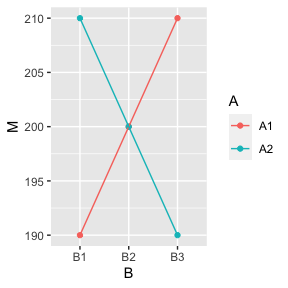# check fixed effects + random effects #summary(lmer(ysim ~ -1 + F + (-1 + F | Subj), data=dat)) summary(lmer(ysim ~ An*(Bn1+Bn2) + (An*(Bn1+Bn2) || Subj), data=dat, control=lmerControl(calc.derivs=FALSE))) ## Linear mixed model fit by REML. t-tests use Satterthwaite's method [ ## lmerModLmerTest] ## Formula: ysim ~ An * (Bn1 + Bn2) + (An * (Bn1 + Bn2) || Subj) ## Data: dat ## Control: lmerControl(calc.derivs = FALSE) ## ## REML criterion at convergence: 3878.9 ## ## Scaled residuals: ## Min 1Q Median 3Q Max ## -2.9981 -0.5290 -0.0416 0.5510 2.4357 ## ## Random effects: ## Groups Name Variance Std.Dev. ## Subj (Intercept) 1030.5065 32.1015 ## Subj.1 An 110.2573 10.5003 ## Subj.2 Bn1 802.6614 28.3313 ## Subj.3 Bn2 414.2810 20.3539 ## Subj.4 An:Bn1 450.3471 21.2214 ## Subj.5 An:Bn2 0.3149 0.5611 ## Residual 2006.3239 44.7920 ## Number of obs: 360, groups: Subj, 60 ## ## Fixed effects: ## Estimate Std. Error df t value Pr(>|t|) ## (Intercept) 2.000e+02 4.770e+00 5.900e+01 41.933 <2e-16 *** ## An 4.518e-16 2.722e+00 5.900e+01 0.000 1.0000 ## Bn1 -1.416e-14 6.842e+00 7.703e+01 0.000 1.0000 ## Bn2 6.642e-15 6.352e+00 7.703e+01 0.000 1.0000 ## An:Bn1 -1.000e+01 6.399e+00 8.530e+01 -1.563 0.1218 ## An:Bn2 -1.000e+01 5.783e+00 6.547e+01 -1.729 0.0885 . ## --- ## Signif. codes: 0 '***' 0.001 '**' 0.01 '*' 0.05 '.' 0.1 ' ' 1 ## ## Correlation of Fixed Effects: ## (Intr) An Bn1 Bn2 An:Bn1 ## An 0.000 ## Bn1 0.000 0.000 ## Bn2 0.000 0.000 -0.385 ## An:Bn1 0.000 0.000 0.000 0.000 ## An:Bn2 0.000 0.000 0.000 0.000 -0.452 aov_car(ysim ~ 1 + Error(Subj/(A*B)), data=dat) ## Anova Table (Type 3 tests) ## ## Response: ysim ## Effect df MSE F ges p.value ## 1 A 1, 59 2667.85 0.00 <.001 >.999 ## 2 B 1.99, 117.12 2931.07 0.00 <.001 >.999 ## 3 A:B 1.92, 113.04 2300.65 5.44 ** .019 .006 ## --- ## Signif. codes: 0 '***' 0.001 '**' 0.01 '*' 0.05 '+' 0.1 ' ' 1 ## ## Sphericity correction method: GG Now, that we saw that our model specification works, we can perform a power analysis. Let’s assume we are mainly interested in the interaction A:B. Because this involves two fixed effects, we perform a model comparison to evaluate their common effect. If you are interested in the specific effect of each of these terms, or in the main effects, similar analyses are possible. nsubj <- seq(10,100,1) # again, we run from 10 to 100 subjects nsim <- length(nsubj) COF <- COFaov <- data.frame() for (i in 1:nsim) { # i <- 1 #print(paste0(i,"/",nsim)) # create experimental design design <- fixed.factor("A", levels=c("A1", "A2")) + fixed.factor("B", levels=c("B1", "B2", "B3")) + random.factor("Subj", instances=nsubj[i]) # set number of subjects dat <- design.codes(design) nsj <- length(unique(dat$Subj))
# set contrasts & compute numeric predictor variables
contrasts(dat$A) <- c(-1, +1) contrasts(dat$B) <- contr.sdif(3)
dat$An <- model.matrix(~A,dat)[,2] dat[,c("Bn1","Bn2")] <- model.matrix(~B,dat)[,2:3] # compute factor F dat$F <- factor(paste0(dat$A, ".", dat$B))
for (j in 1:4) { # j <- 1 # run 4 models for each number of subjects
# simulate data
dat$ysim <- simLMM(form, data=dat, Fixef=fix, VC_sd=list(sd_Subj, sd_Res), CP=0.85, empirical=FALSE, verbose=FALSE) # ANOVA AOV <- aov_car(ysim ~ 1 + Error(Subj/(A*B)), data=dat) AOVcof <- summary(AOV)$univariate.tests[4,]
COFaov <- rbind(COFaov,c(nsj,AOVcof))
# LMM: perform model comparison
LMM1 <- lmer(ysim ~ An*(Bn1+Bn2) + (An*(Bn1+Bn2) || Subj), data=dat, REML=FALSE, control=lmerControl(calc.derivs=FALSE))
LMM0 <- lmer(ysim ~ An+(Bn1+Bn2) + (An*(Bn1+Bn2) || Subj), data=dat, REML=FALSE, control=lmerControl(calc.derivs=FALSE))
LMMcof <- c(coef(summary(LMM1))[5:6,5],
as.numeric(data.frame(anova(LMM1,LMM0))[2,6:8]))
COF <- rbind(COF,c(nsj,LMMcof,isSingular(LMM1) & isSingular(LMM0)))
}
}
# results from LMMs
names(COF) <- c("nsj","p_An:Bn1","p_An:Bn2","Chisq","Df","p","singular")
COF$sign <- as.numeric(COF$p < .05)
COF$nsjF <- gtools::quantcut(COF$nsj, q=seq(0,1,length=10))
COF$nsjFL <- plyr::ddply(COF, "nsjF", transform, nsjFL=mean(nsj))$nsjFL
# results for ANOVA
COFaov$sign <- as.numeric(COFaov$p < .05)
COFaov$nsjF <- gtools::quantcut(COFaov$nsj, q=seq(0,1,length=10))
COFaov$nsjFL <- plyr::ddply(COFaov,"nsjF",transform,nsjFL=mean(nsj))$nsjFL
#save(COF, COFaov, file="data/Power1.rda")

Note that we here compute power only for the interaction term A:B. We actually assumed in the simulations that the main effects are exactly zero. However, equivalent power analyses are also possible for main effects.

Again, we first check how many fits were singular:

load(file=system.file("power-analyses", "Power1.rda", package = "designr"))
mean(COF$singular) ##  0.6703297 Here, we see that there is a large number of singular fits: roughly 70% of all model fits were singular suggesting that the random effects structure used in the model was not fully supported by the data. Opinions differ on whether this is a problem and how to deal with this. This paper, for example argues that singular fits can be ignored (https://debruine.github.io/lmem_sim/articles/paper.html). However, we would argue that sometimes singular fits can be problematic (in particular when one is interested in the random effects). Of course, convergence problems should also be monitored in addition. p1 <- ggplot(data=COF)+ geom_smooth(aes(x=nsj, y=sign))+ geom_point( stat="summary", aes(x=nsjFL, y=sign))+ geom_errorbar(stat="summary", aes(x=nsjFL, y=sign))+ geom_line( stat="summary", aes(x=nsjFL, y=sign)) p2 <- ggplot(data=COFaov)+ geom_smooth(aes(x=nsj, y=sign))+ geom_point( stat="summary", aes(x=nsjFL, y=sign))+ geom_errorbar(stat="summary", aes(x=nsjFL, y=sign))+ geom_line( stat="summary", aes(x=nsjFL, y=sign)) gridExtra::grid.arrange(p1,p2, nrow=1)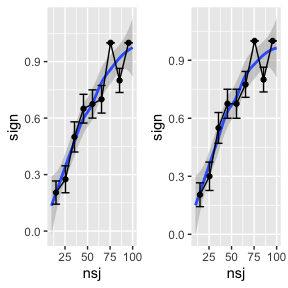Determine number of subjects needed for a power of 80%. m0 <- loess(sign ~ nsj, data=COF) COF$pred <- predict(m0)
idx <- COF$pred>0.8 min(COF$nsj[idx])
##  67

# 6 LMM: Crossed random effects for subjects and items

Let’s examine a design with only one factor X, but with crossed random effects for subjects and items, where factor X is a within-subject and between-item factor.

The computations are quite straightforward. Note that we now assume 3 random effects terms, one for subjects, one for items, and one residual noise term.

design <-
fixed.factor("X", levels=c("X1", "X2")) +
random.factor("Subj", instances=15) +
random.factor("Item", groups="X", instances=2)
dat <- design.codes(design)
(contrasts(dat$X) <- c(-1, +1)) ##  -1 1 dat$Xn <- model.matrix(~X,dat)[,2]

# simulate data
fix     <- c(200, 5) # set fixed effects
sd_Subj <- c(30, 10) # set random effects standard deviations for subjects
sd_Item <- c(10)     # set random effects standard deviations for items
sd_Res  <- 50        # set residual standard deviation
dat$ysim <- simLMM(form=~ X + (X | Subj) + (1 | Item), data=dat, Fixef=fix, VC_sd=list(sd_Subj, sd_Item, sd_Res), CP=0.3, empirical=TRUE) ## Data simulation from a linear mixed-effects model (LMM) ## Formula: gaussian ~ 1 + X1 + ( 1 + X1 | Subj ) + ( 1 | Item ) ## empirical = TRUE ## Random effects: ## Groups Name Std.Dev. Corr ## Subj (Intercept) 30.0 ## X1 10.0 0.30 ## Item (Intercept) 10.0 ## Residual 50.0 ## Number of obs: 60, groups: Subj, 15; Item, 4 ## Fixed effects: ## (Intercept) X1 ## 200 5 # check group means dat %>% group_by(X) %>% summarize(M=mean(ysim)) ## # A tibble: 2 × 2 ## X M ## <fct> <dbl> ## 1 X1 195 ## 2 X2 205 # check fixed effects + random effects summary(lmer(ysim ~ Xn + (Xn || Subj) + (1 | Item), data=dat, control=lmerControl(calc.derivs=FALSE))) ## Linear mixed model fit by REML. t-tests use Satterthwaite's method [ ## lmerModLmerTest] ## Formula: ysim ~ Xn + (Xn || Subj) + (1 | Item) ## Data: dat ## Control: lmerControl(calc.derivs = FALSE) ## ## REML criterion at convergence: 644.3 ## ## Scaled residuals: ## Min 1Q Median 3Q Max ## -1.4539 -0.6250 -0.1323 0.6764 1.8460 ## ## Random effects: ## Groups Name Variance Std.Dev. ## Subj (Intercept) 1171.4 34.23 ## Subj.1 Xn 246.3 15.69 ## Item (Intercept) 0.0 0.00 ## Residual 2406.2 49.05 ## Number of obs: 60, groups: Subj, 15; Item, 4 ## ## Fixed effects: ## Estimate Std. Error df t value Pr(>|t|) ## (Intercept) 200.000 10.872 14.000 18.396 3.33e-11 *** ## Xn 5.000 7.518 14.000 0.665 0.517 ## --- ## Signif. codes: 0 '***' 0.001 '**' 0.01 '*' 0.05 '.' 0.1 ' ' 1 ## ## Correlation of Fixed Effects: ## (Intr) ## Xn 0.000 ## optimizer (nloptwrap) convergence code: 0 (OK) ## boundary (singular) fit: see help('isSingular') In the power analysis, we again continuously increase the number of subjects. An alternative or additional analysis could involve continuously increasing the number of items and testing how many items are needed to achieve a certain power. nsubj <- seq(10,100,4) nsimSj <- length(nsubj) nitem <- seq(2,30,4) nsimIt <- length(nitem) COF <- data.frame() for (i in 1:nsimSj) { # i <- 1 #print(paste0(i,"/",nsimSj)) for (j in 1:nsimIt) { # j <- 1 design <- fixed.factor("X", levels=c("X1", "X2")) + random.factor("Subj", instances=nsubj[i]) + random.factor("Item", groups="X", instances=nitem[j]) dat <- design.codes(design) nsj <- length(unique(dat$Subj))
nit <- length(unique(dat$Item)) contrasts(dat$X) <- c(-1, +1)
dat$Xn <- model.matrix(~X,dat)[,2] for (j in 1:5) { # j <- 1 dat$ysim <- simLMM(~ X + (X | Subj) + (1 | Item), data=dat,
Fixef=fix, VC_sd=list(sd_Subj, sd_Item, sd_Res), CP=0.3,
empirical=FALSE, verbose=FALSE)
LMM <- lmer(ysim ~ Xn + (Xn || Subj) + (1 | Item), data=dat, REML=FALSE,
control=lmerControl(calc.derivs=FALSE))
LMMcof <- coef(summary(LMM))[2,]
COF <- rbind(COF,c(nsj,nit,LMMcof, isSingular(LMM)))
}
}
}
# results for LMM
names(COF)<- c("nsj","nit","Estimate","SE","df","t","p","singular")
COF$sign <- as.numeric(COF$p < .05 & COF$Estimate>0) COF$nsjF  <- gtools::quantcut(COF$nsj, q=seq(0,1,length=10)) COF$nsjFL <- plyr::ddply(COF,"nsjF",transform,nsjFL=mean(nsj))$nsjFL COF$nitF  <- gtools::quantcut(COF$nit, q=seq(0,1,length=5)) COF$nitFL <- plyr::ddply(COF,"nitF",transform,nitFL=mean(nsj))$nitFL #save(COF, file="data/Power2.rda") Again, we plot the power as a function of the number of subjects. We see that power increases with increasing numbers of subjects and with increasing the numbers of items. load(file=system.file("power-analyses", "Power2.rda", package = "designr")) ggplot(data=COF) + geom_smooth(aes(x=nsj, y=sign, colour=factor(nitF)), se=FALSE)+ geom_point( stat="summary", aes(x=nsjFL, y=sign, colour=factor(nitF)))+ geom_errorbar(stat="summary", aes(x=nsjFL, y=sign, colour=factor(nitF)))+ geom_line( stat="summary", aes(x=nsjFL, y=sign, colour=factor(nitF)))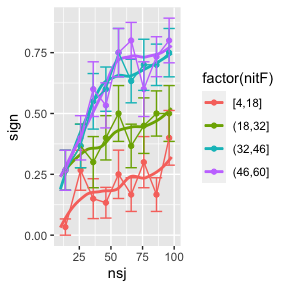# determine number of subjects needed for a power of 60% idx <- which(COF$nit>46)
m0 <- loess(sign ~ nsj, data=COF[idx,])
COF$pred <- NA COF$pred[idx] <- predict(m0)
min(COF$nsj[COF$pred>0.5], na.rm=TRUE)
##  38

# 7 Vary effect size

One important aspect in power analyses is that we have to assume the true effect is known. However, we are usually unsure about its true value. Therefore, it can make sense to assume different values for the effect size and to look at how this impacts on power.

design <-
fixed.factor("X", levels=c("X1", "X2")) +
random.factor("Subj", instances=15) +
random.factor("Item", groups="X", instances=4)
dat <- design.codes(design)
length(unique(dat$Subj)) ##  15 length(unique(dat$Item))
##  8
(contrasts(dat$X) <- c(-1, +1)) ##  -1 1 dat$Xn <- model.matrix(~X,dat)[,2]

fix      <- c(200,10)
sd_Subj  <- c(30, 10)
sd_Item  <- c(10)
sd_Res   <- 50
dat$ysim <- simLMM(~ X + (X | Subj) + (1 | Item), data=dat, Fixef=fix, VC_sd=list(sd_Subj, sd_Item, sd_Res), CP=0.3, empirical=TRUE) ## Data simulation from a linear mixed-effects model (LMM) ## Formula: gaussian ~ 1 + X1 + ( 1 + X1 | Subj ) + ( 1 | Item ) ## empirical = TRUE ## Random effects: ## Groups Name Std.Dev. Corr ## Subj (Intercept) 30.0 ## X1 10.0 0.30 ## Item (Intercept) 10.0 ## Residual 50.0 ## Number of obs: 120, groups: Subj, 15; Item, 8 ## Fixed effects: ## (Intercept) X1 ## 200 10 dat %>% group_by(X) %>% summarize(M=mean(ysim)) ## # A tibble: 2 × 2 ## X M ## <fct> <dbl> ## 1 X1 190 ## 2 X2 210 lmer(ysim ~ Xn + (Xn || Subj) + (1 | Item), data=dat, control=lmerControl(calc.derivs=FALSE)) ## Linear mixed model fit by REML ['lmerModLmerTest'] ## Formula: ysim ~ Xn + (Xn || Subj) + (1 | Item) ## Data: dat ## REML criterion at convergence: 1288.898 ## Random effects: ## Groups Name Std.Dev. ## Subj (Intercept) 26.37 ## Subj.1 Xn 16.81 ## Item (Intercept) 14.08 ## Residual 47.78 ## Number of obs: 120, groups: Subj, 15; Item, 8 ## Fixed Effects: ## (Intercept) Xn ## 200 10 The data simulations look good. We next go to the power simulations. nsubj <- seq(10,100,1) nsim <- length(nsubj) COF <- data.frame() for (i in 1:nsim) { #print(paste0(i,"/",nsim)) design <- fixed.factor("X", levels=c("X1", "X2")) + random.factor("Subj", instances=nsubj[i]) + random.factor("Item", groups="X", instances=4) dat <- design.codes(design) nsj <- length(unique(dat$Subj))
contrasts(dat$X) <- c(-1, +1) dat$Xn <- model.matrix(~X,dat)[,2]
for (j in 1:18) {
# assume three different effect sizes for the fixed effect: 5, 10, or 15
FIX <- c(5,10,15)
fix <- c(200,FIX[(j%%3)+1])
dat$ysim <- simLMM(~ X + (X | Subj) + (1 | Item), data=dat, Fixef=fix, VC_sd=list(sd_Subj, sd_Item, sd_Res), CP=0.3, empirical=FALSE, verbose=FALSE) LMM <- lmer(ysim ~ Xn + (Xn || Subj) + (1 | Item), data=dat, control=lmerControl(calc.derivs=FALSE)) LMMcof <- coef(summary(LMM))[2,] COF <- rbind(COF,c(nsj,fix,LMMcof)) # store effect size } } # results for LMM names(COF) <- c("nsj","EffectSize","Estimate","SE","df","t","p") COF$sign <- as.numeric(COF$p < .05 & COF$Estimate>0)
COF$nsjF <- gtools::quantcut(COF$nsj, q=seq(0,1,length=10))
COF$nsjFL <- plyr::ddply(COF,"nsjF",transform,nsjFL=mean(nsj))$nsjFL
#save(COF, file="data/Power3.rda")
load(file=system.file("power-analyses", "Power3.rda", package = "designr"))
ggplot(data=COF) +
geom_smooth(aes(x=nsj, y=sign, colour=factor(EffectSize)))+
geom_point(stat="summary", aes(x=nsjFL, y=sign, colour=factor(EffectSize)))+
geom_errorbar(stat="summary", aes(x=nsjFL, y=sign, colour=factor(EffectSize)))+
geom_line(stat="summary", aes(x=nsjFL, y=sign, colour=factor(EffectSize)))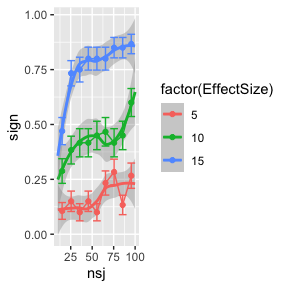The results show that power strongly varies as a function of the assumed effect size. This is something important to consider: maybe we are not really sure about the power of our experiment.

Determine number of subjects needed for a power of 50% under the assumption of an effect size of middle size (i.e., fixed effect of 10).

m0 <- loess(sign ~ nsj, data=subset(COF,EffectSize==10))
COF$pred <- NA COF$pred[COF$EffectSize==10] <- predict(m0) idx <- COF$pred>0.5
min(COF$nsj[idx], na.rm=TRUE) # 70 ##  88 # 8 Based on a previous data set: latin square design Now let’s consider the case that we want to use results from a prior experiment as the assumption about effect sizes in our power analysis. First we load the data from the prior study. # load empirical data data(gibsonwu2013) # the Gibson & Wu (2013) dataset is included in the designr package gw1 <- subset(gibsonwu2013, region=="headnoun") # subset critical region gw1$so <- ifelse(gw1$type %in% c("subj-ext"),-1,1) # sum-coding for predictor dat2 <- gw1[,c("subj","item","so","type2","rt")] # extract experimental design datE <- dplyr::arrange(dat2, subj, item) length(unique(datE$subj)) # 37
##  37
length(unique(datE$item)) # 15 ##  15 # we re-create the empirical design from the experiment using designr design <- fixed.factor("X", levels=c("X1", "X2")) + random.factor("subj", instances=18) + random.factor("item", instances= 8) + random.factor(c("subj", "item"), groups=c("X")) dat <- dplyr::arrange(design.codes(design), subj, item) (contrasts(dat$X) <- c(+1, -1))
##   1 -1
dat$so <- model.matrix(~X,dat)[,2] length(unique(dat$subj)) # 36
##  36
length(unique(dat$item)) # 16 ##  16 # compare designs head(datE,20) ## subj item so type2 rt ## 2001 1 1 1 object relative 246 ## 1192 1 2 -1 subject relative 542 ## 1591 1 3 1 object relative 406 ## 753 1 4 -1 subject relative 286 ## 341 1 5 1 object relative 582 ## 221 1 6 -1 subject relative 959 ## 1471 1 7 1 object relative 270 ## 922 1 8 -1 subject relative 438 ## 461 1 9 1 object relative 294 ## 1054 1 10 -1 subject relative 278 ## 1342 1 11 1 object relative 494 ## 94 1 13 1 object relative 1561 ## 621 1 14 -1 subject relative 438 ## 1891 1 15 1 object relative 286 ## 1761 1 16 -1 subject relative 374 ## 20371 2 1 -1 subject relative 286 ## 19801 2 2 1 object relative 278 ## 19501 2 3 -1 subject relative 254 ## 20521 2 4 1 object relative 734 ## 18991 2 5 -1 subject relative 390 head(data.frame(dat),20) ## subj item X so ## 1 subj01 item01 X1 1 ## 2 subj01 item02 X2 -1 ## 3 subj01 item03 X1 1 ## 4 subj01 item04 X2 -1 ## 5 subj01 item05 X1 1 ## 6 subj01 item06 X2 -1 ## 7 subj01 item07 X1 1 ## 8 subj01 item08 X2 -1 ## 9 subj01 item09 X1 1 ## 10 subj01 item10 X2 -1 ## 11 subj01 item11 X1 1 ## 12 subj01 item12 X2 -1 ## 13 subj01 item13 X1 1 ## 14 subj01 item14 X2 -1 ## 15 subj01 item15 X1 1 ## 16 subj01 item16 X2 -1 ## 17 subj02 item01 X2 -1 ## 18 subj02 item02 X1 1 ## 19 subj02 item03 X2 -1 ## 20 subj02 item04 X1 1 tail(datE,20) ## subj item so type2 rt ## 59661 39 11 1 object relative 2053 ## 59151 39 13 1 object relative 445 ## 60061 39 14 -1 subject relative 222 ## 59951 39 15 1 object relative 430 ## 58901 39 16 -1 subject relative 302 ## 63041 40 1 -1 subject relative 422 ## 64031 40 2 1 object relative 318 ## 63191 40 3 -1 subject relative 421 ## 62871 40 4 1 object relative 462 ## 64301 40 5 -1 subject relative 1262 ## 64551 40 6 1 object relative 438 ## 64181 40 7 -1 subject relative 356 ## 63761 40 8 1 object relative 1509 ## 63471 40 9 -1 subject relative 390 ## 63891 40 10 1 object relative 358 ## 64801 40 11 -1 subject relative 390 ## 64421 40 13 -1 subject relative 318 ## 64671 40 14 1 object relative 278 ## 63361 40 15 -1 subject relative 461 ## 63631 40 16 1 object relative 294 tail(data.frame(dat),20) ## subj item X so ## 557 subj35 item13 X1 1 ## 558 subj35 item14 X2 -1 ## 559 subj35 item15 X1 1 ## 560 subj35 item16 X2 -1 ## 561 subj36 item01 X2 -1 ## 562 subj36 item02 X1 1 ## 563 subj36 item03 X2 -1 ## 564 subj36 item04 X1 1 ## 565 subj36 item05 X2 -1 ## 566 subj36 item06 X1 1 ## 567 subj36 item07 X2 -1 ## 568 subj36 item08 X1 1 ## 569 subj36 item09 X2 -1 ## 570 subj36 item10 X1 1 ## 571 subj36 item11 X2 -1 ## 572 subj36 item12 X1 1 ## 573 subj36 item13 X2 -1 ## 574 subj36 item14 X1 1 ## 575 subj36 item15 X2 -1 ## 576 subj36 item16 X1 1 # transform dependent variable hist(datE$rt)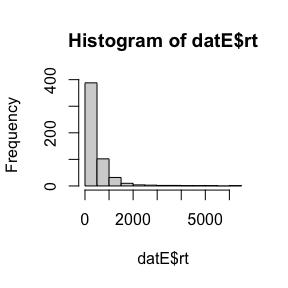boxcox(rt ~ so, data=datE)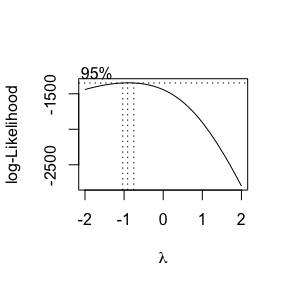datE$speed <- 1/datE$rt
hist(datE$speed)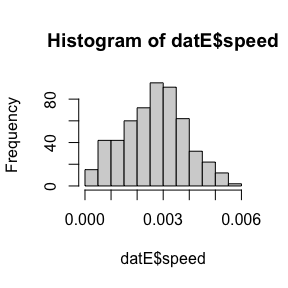qqnorm(datE$speed); qqline(datE$speed)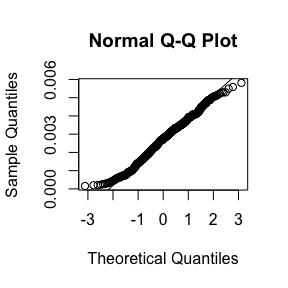# run LMM on speed variable lmm <- lmer(speed ~ so + (so|subj) + (so|item), data=datE, control=lmerControl(calc.derivs=FALSE)) ## boundary (singular) fit: see help('isSingular') summary(lmm) ## Linear mixed model fit by REML. t-tests use Satterthwaite's method [ ## lmerModLmerTest] ## Formula: speed ~ so + (so | subj) + (so | item) ## Data: datE ## Control: lmerControl(calc.derivs = FALSE) ## ## REML criterion at convergence: -5932.5 ## ## Scaled residuals: ## Min 1Q Median 3Q Max ## -3.2501 -0.5996 0.1237 0.6430 2.5441 ## ## Random effects: ## Groups Name Variance Std.Dev. Corr ## subj (Intercept) 3.712e-07 6.093e-04 ## so 1.331e-08 1.154e-04 -0.51 ## item (Intercept) 1.100e-07 3.317e-04 ## so 2.305e-09 4.801e-05 1.00 ## Residual 8.916e-07 9.442e-04 ## Number of obs: 547, groups: subj, 37; item, 15 ## ## Fixed effects: ## Estimate Std. Error df t value Pr(>|t|) ## (Intercept) 2.672e-03 1.379e-04 3.806e+01 19.369 <2e-16 *** ## so 3.879e-05 4.644e-05 2.966e+01 0.835 0.41 ## --- ## Signif. codes: 0 '***' 0.001 '**' 0.01 '*' 0.05 '.' 0.1 ' ' 1 ## ## Correlation of Fixed Effects: ## (Intr) ## so 0.012 ## optimizer (nloptwrap) convergence code: 0 (OK) ## boundary (singular) fit: see help('isSingular') # the random effects correlation for items is estimated as 1, indicating a singular fit lmm <- lmer(speed ~ so + (so|subj) + (so||item), data=datE, control=lmerControl(calc.derivs=FALSE)) ## boundary (singular) fit: see help('isSingular') summary(lmm) ## Linear mixed model fit by REML. t-tests use Satterthwaite's method [ ## lmerModLmerTest] ## Formula: speed ~ so + (so | subj) + (so || item) ## Data: datE ## Control: lmerControl(calc.derivs = FALSE) ## ## REML criterion at convergence: -5931.3 ## ## Scaled residuals: ## Min 1Q Median 3Q Max ## -3.1531 -0.5908 0.1328 0.6442 2.5066 ## ## Random effects: ## Groups Name Variance Std.Dev. Corr ## subj (Intercept) 3.718e-07 6.098e-04 ## so 1.315e-08 1.147e-04 -0.51 ## item (Intercept) 1.098e-07 3.314e-04 ## item.1 so 1.809e-15 4.253e-08 ## Residual 8.941e-07 9.456e-04 ## Number of obs: 547, groups: subj, 37; item, 15 ## ## Fixed effects: ## Estimate Std. Error df t value Pr(>|t|) ## (Intercept) 2.672e-03 1.379e-04 3.807e+01 19.371 <2e-16 *** ## so 3.810e-05 4.477e-05 3.553e+01 0.851 0.4 ## --- ## Signif. codes: 0 '***' 0.001 '**' 0.01 '*' 0.05 '.' 0.1 ' ' 1 ## ## Correlation of Fixed Effects: ## (Intr) ## so -0.159 ## optimizer (nloptwrap) convergence code: 0 (OK) ## boundary (singular) fit: see help('isSingular') Based on these analyses, we take the last fitted lmm model, and use this to simulate new data. This is possible with the simple function call simLMM(LMM=lmm). This uses the data that the model was fit on. However, we can also use the fit lmm object to simulate data for a new data set (i.e., here the experimental design we created we created using designr), by simply adding the new data frame: simLMM(data=dat, LMM=lmm). Importantly, the new data frame needs to have the variables that are used in the model. datE$simSpeed <- simLMM(LMM=lmm, empirical=TRUE)
## Data simulation from a linear mixed-effects model (LMM)
## Formula: gaussian ~ 1 + so + ( 1 + so | subj ) + ( 1 | item ) + ( 0 + so | item )
## empirical = TRUE
## Random effects:
##  Groups    Name          Std.Dev.      Corr
##  subj      (Intercept)   0.0006098
##            so            0.0001147     -0.51
##  item      (Intercept)   0.0003314
##  item      so            0.0000000
##  Residual                0.0009456
## Number of obs: 547, groups:  subj, 37; item, 15
## Fixed effects:
##  (Intercept)           so
## 2.672153e-03 3.809511e-05
dat$speed <- simLMM(data=dat, LMM=lmm, empirical=TRUE) ## Data simulation from a linear mixed-effects model (LMM) ## Formula: gaussian ~ 1 + so + ( 1 + so | subj ) + ( 1 | item ) + ( 0 + so | item ) ## empirical = TRUE ## Random effects: ## Groups Name Std.Dev. Corr ## subj (Intercept) 0.0006098 ## so 0.0001147 -0.51 ## item (Intercept) 0.0003314 ## item so 0.0000000 ## Residual 0.0009456 ## Number of obs: 576, groups: subj, 36; item, 16 ## Fixed effects: ## (Intercept) so ## 2.672153e-03 3.809511e-05 dat %>% group_by(so) %>% summarize(M=mean(speed)) ## # A tibble: 2 × 2 ## so M ## <dbl> <dbl> ## 1 -1 0.00263 ## 2 1 0.00271 lmer(speed ~ so + (so||subj) + (so||item), data=dat, control=lmerControl(calc.derivs=FALSE)) ## boundary (singular) fit: see help('isSingular') ## Linear mixed model fit by REML ['lmerModLmerTest'] ## Formula: speed ~ so + (so || subj) + (so || item) ## Data: dat ## REML criterion at convergence: -6250.4 ## Random effects: ## Groups Name Std.Dev. ## subj (Intercept) 5.353e-04 ## subj.1 so 3.669e-05 ## item (Intercept) 3.383e-04 ## item.1 so 0.000e+00 ## Residual 9.565e-04 ## Number of obs: 576, groups: subj, 36; item, 16 ## Fixed Effects: ## (Intercept) so ## 0.0026722 0.0000381 ## optimizer (nloptwrap) convergence code: 0 (OK) ; 0 optimizer warnings; 1 lme4 warnings The results show that we can exactly recover the parameters from the previous model in the new simulated data set. Next, we can turn to perform power analyses. # perform power analysis based on fitted model nsubj <- seq(10,100,1) nsim <- length(nsubj) COF <- data.frame() for (i in 1:nsim) { # i <- 1 #print(paste0(i,"/",nsim)) design <- fixed.factor("X", levels=c("X1", "X2")) + random.factor("subj", instances=nsubj[i]) + random.factor("item", instances= 8) + random.factor(c("subj", "item"), groups=c("X")) dat <- dplyr::arrange(design.codes(design), subj, item) contrasts(dat$X) <- c(+1, -1)
dat$so <- model.matrix(~X,dat)[,2] nsj <- length(unique(dat$subj))
for (j in 1:10) { # j <- 1
dat$speed <- simLMM(data=dat, LMM=lmm, empirical=FALSE, verbose=FALSE) LMMcof <- coef(summary(lmer(speed ~ so + (so||subj) + (so||item), data=dat, control=lmerControl(calc.derivs=FALSE))))[2,] COF <- rbind(COF,c(nsj,LMMcof)) } } names(COF) <- c("nsj","Estimate","SE","df","t","p") COF$sign <- as.numeric(COF$p < .05 & COF$Estimate>0)
COF$nsjF <- gtools::quantcut(COF$nsj, q=seq(0,1,length=10))
COF$nsjFL <- plyr::ddply(COF,"nsjF",transform,nsjFL=mean(nsj))$nsjFL
#save(COF, file="data/Power4.rda")
load(file=system.file("power-analyses", "Power4.rda", package = "designr"))
ggplot(data=COF) +
geom_smooth(aes(x=nsj, y=sign))+
geom_point(stat="summary", aes(x=nsjFL, y=sign))+
geom_errorbar(stat="summary", aes(x=nsjFL, y=sign))+
geom_line(stat="summary", aes(x=nsjFL, y=sign))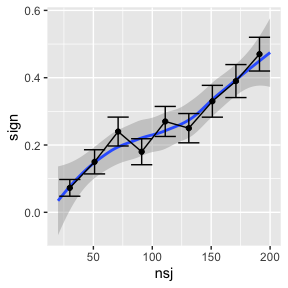fixef(lmm)
##  (Intercept)           so
## 2.672153e-03 3.809511e-05
mean(COF$Estimate) ##  3.859278e-05 # determine number of subjects needed for a power of 40% m0 <- loess(sign ~ nsj, data=COF) COF$pred <- predict(m0)
idx <- COF$pred>0.4 min(COF$nsj[idx]) # 164
##  174

We can see that the power for this study is quite low. Notice that we are using a realistic effect size estimate from a previous study. Yet, even 200 subjects are not sufficient to obtain a power of 80% to detect the true effect.

# 9 LMM: Crossed random effects for subjects and items - with continuous covariate

Next, we treat the case of a continuous covariate. Interestingly, we can use simLMM to simulate not only a dependent variable, but also a covariate.

design <-
fixed.factor("X", levels=c("X1", "X2")) +
random.factor("Subj", instances=15) +
random.factor("Item", groups="X", instances=10)
dat <- design.codes(design)
(contrasts(dat$X) <- c(-1, +1)) ##  -1 1 dat$Xn <- model.matrix(~X,dat)[,2]

simLMM has a further argument: family. This can be set to “lp”, which stands for “linear predictor”. This means that simLMM does not add any residual noise, but puts out the best prediction for each data point. Using this functionality, we can generate covariates that only differ by subject (i.e., age in the example below), or covariates that only differ by item (i.e., word frequency in the example below). However, we can also simulate a covariate that varies across subjects, items, and across individual trials. For example, when modeling fixation durations during reading, we might include the previous fixation duration as a predictor (covariate). When modeling ratings, we might have a task where each trial has two ratings, and we want to predict the second rating using the first as predictor (covariate). However, these differences are mainly important for simulating the covariate.

# simulate covariate
dat$age <- round( simLMM(form=~ 1 + (1 | Subj), data=dat, Fixef=30, VC_sd=list(5), empirical=TRUE, family="lp") ) ## Data simulation from a linear mixed-effects model (LMM): linear predictor ## Formula: lp ~ 1 + ( 1 | Subj ) ## empirical = TRUE ## Random effects: ## Groups Name Std.Dev.Corr ## Subj (Intercept) 5.0 ## Number of obs: 300, groups: Subj, 15 ## Fixed effects: ## (Intercept) ## 30 #table(dat$Subj,dat$age) hist(dat$age)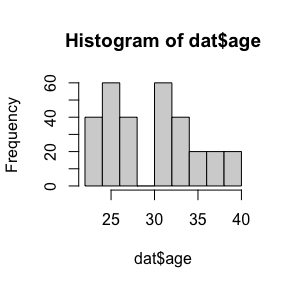dat$wordFrequency <- round( simLMM(form=~ 1 + (1 | Item), data=dat, Fixef=4, VC_sd=list(1), empirical=TRUE, family="lp") ) ## Data simulation from a linear mixed-effects model (LMM): linear predictor ## Formula: lp ~ 1 + ( 1 | Item ) ## empirical = TRUE ## Random effects: ## Groups Name Std.Dev.Corr ## Item (Intercept) 1.0 ## Number of obs: 300, groups: Item, 20 ## Fixed effects: ## (Intercept) ## 4 #table(dat$Item,dat$wordFrequency) hist(dat$wordFrequency)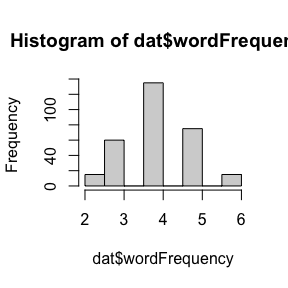fix     <- c(5) # set fixed effects
sd_Subj <- c(1) # set random effects standard deviations for subjects
sd_Item <- c(1) # set random effects standard deviations for items
sd_Res  <- 1    # set residual standard deviation
dat$rating <- round( simLMM(form=~ 1 + (1 | Subj) + (1 | Item), data=dat, Fixef=fix, VC_sd=list(sd_Subj, sd_Item, sd_Res), empirical=TRUE) ) ## Data simulation from a linear mixed-effects model (LMM) ## Formula: gaussian ~ 1 + ( 1 | Subj ) + ( 1 | Item ) ## empirical = TRUE ## Random effects: ## Groups Name Std.Dev.Corr ## Subj (Intercept) 1.0 ## Item (Intercept) 1.0 ## Residual 1.0 ## Number of obs: 300, groups: Subj, 15; Item, 20 ## Fixed effects: ## (Intercept) ## 5 hist(dat$rating)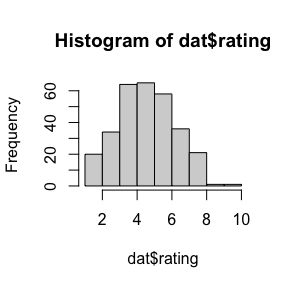dat$rating.c <- dat$rating - mean(dat$rating) Note that we mean-center the covariate. Importantly, when we use a covariate (i.e., continuous variable), then it is not possible to use empirical=TRUE. We have to switch to empirical=FALSE. # simulate data fix <- c(200,20, 7, 5) # set fixed effects sd_Subj <- c(30, 10, 5, 5) # set random effects standard deviations for subjects sd_Item <- c(10) # set random effects standard deviations for items sd_Res <- 50 # set residual standard deviation dat$ysim <- simLMM(form=~ X*rating.c + (X*rating.c | Subj) + (1 | Item), data=dat,
Fixef=fix, VC_sd=list(sd_Subj, sd_Item, sd_Res), CP=0.3,
empirical=FALSE)
## Data simulation from a linear mixed-effects model (LMM)
## Formula: gaussian ~ 1 + X1 + rating.c + X1:rating.c + ( 1 + X1 + rating.c + X1:rating.c | Subj ) + ( 1 | Item )
## empirical = FALSE
## Random effects:
##  Groups    Name          Std.Dev. Corr
##  Subj      (Intercept)   30.0
##            X1            10.0     0.30
##            rating.c       5.0     0.30   0.30
##            X1:rating.c    5.0     0.30   0.30   0.30
##  Item      (Intercept)   10.0
##  Residual                50.0
## Number of obs: 300, groups:  Subj, 15; Item, 20
## Fixed effects:
## (Intercept)          X1    rating.c X1:rating.c
##         200          20           7           5
# check group means
dat %>% group_by(X) %>% summarize(M=mean(ysim))
## # A tibble: 2 × 2
##   X         M
##   <fct> <dbl>
## 1 X1     174.
## 2 X2     228.
# check fixed effects + random effects
summary(lmer(ysim ~ Xn*rating.c + (Xn*rating.c || Subj) + (1 | Item), data=dat, control=lmerControl(calc.derivs=FALSE)))
## boundary (singular) fit: see help('isSingular')
## Linear mixed model fit by REML. t-tests use Satterthwaite's method [
## lmerModLmerTest]
## Formula: ysim ~ Xn * rating.c + (Xn * rating.c || Subj) + (1 | Item)
##    Data: dat
## Control: lmerControl(calc.derivs = FALSE)
##
## REML criterion at convergence: 3224.8
##
## Scaled residuals:
##      Min       1Q   Median       3Q      Max
## -2.95068 -0.61584 -0.03999  0.65256  2.74467
##
## Random effects:
##  Groups   Name        Variance Std.Dev.
##  Item     (Intercept)  173.637 13.177
##  Subj     Xn:rating.c    4.919  2.218
##  Subj.1   rating.c       0.000  0.000
##  Subj.2   Xn             0.000  0.000
##  Subj.3   (Intercept)  655.056 25.594
##  Residual             2545.620 50.454
## Number of obs: 300, groups:  Item, 20; Subj, 15
##
## Fixed effects:
##             Estimate Std. Error      df t value Pr(>|t|)
## (Intercept)  201.946      7.808  16.951  25.863 4.63e-15 ***
## Xn            29.072      4.195  16.404   6.930 2.96e-06 ***
## rating.c       9.183      2.346 132.621   3.915 0.000144 ***
## Xn:rating.c    6.205      2.039  12.925   3.043 0.009480 **
## ---
## Signif. codes:  0 '***' 0.001 '**' 0.01 '*' 0.05 '.' 0.1 ' ' 1
##
## Correlation of Fixed Effects:
##             (Intr) Xn    rtng.c
## Xn          0.000
## rating.c    0.001  0.095
## Xn:rating.c 0.041  0.007 0.014
## optimizer (nloptwrap) convergence code: 0 (OK)
## boundary (singular) fit: see help('isSingular')
nsubj <- seq(10,100,1)
nsim <- length(nsubj)
COF <- data.frame()
for (i in 1:nsim) { # i <- 1
#print(paste0(i,"/",nsim))
design <-
fixed.factor("X", levels=c("X1", "X2")) +
random.factor("Subj", instances=nsubj[i]) +
random.factor("Item", groups="X", instances=2)
dat <- design.codes(design)
nsj <- length(unique(dat$Subj)) contrasts(dat$X) <- c(-1, +1)
dat$Xn <- model.matrix(~X,dat)[,2] for (j in 1:10) { # j <- 1 dat$rating <- round( simLMM(form=~ 1 + (1 | Subj) + (1 | Item), data=dat,
Fixef=5, VC_sd=list(1, 1, 1), empirical=FALSE, verbose=FALSE) )
dat$rating.c <- dat$rating - mean(dat$rating) fix <- c(200,20, 7, 5) # set fixed effects sd_Subj <- c(30, 10, 5, 5) # set random effects standard deviations for subjects sd_Item <- c(10) # set random effects standard deviations for items sd_Res <- 50 # set residual standard deviation dat$ysim <- simLMM(form=~ X*rating.c + (X*rating.c | Subj) + (1 | Item), data=dat,
Fixef=fix, VC_sd=list(sd_Subj, sd_Item, sd_Res), CP=0.3,
empirical=FALSE, verbose=FALSE)
LMM <- lmer(ysim ~ Xn*rating.c + (Xn*rating.c || Subj) + (1 | Item), data=dat,
control=lmerControl(calc.derivs=FALSE))
LMMcof <- coef(summary(LMM))[4,] # extract the stats for the interaction
COF <- rbind(COF,c(nsj,LMMcof))
}
}
names(COF) <- c("nsj","Estimate","SE","df","t","p")
COF$sign <- as.numeric(COF$p < .05 & COF$Estimate>0) COF$nsjF  <- gtools::quantcut(COF$nsj, q=seq(0,1,length=10)) COF$nsjFL <- plyr::ddply(COF,"nsjF",transform,nsjFL=mean(nsj))$nsjFL #save(COF, file="data/Power5.rda") We are here only interested in the interaction between the covariate and the factor, i.e., whether the slopes differ between conditions. Of course, one could also study the main effects. load(file=system.file("power-analyses", "Power5.rda", package = "designr")) ggplot(data=COF) + geom_smooth(aes(x=nsj, y=sign))+ geom_point( stat="summary", aes(x=nsjFL, y=sign))+ geom_errorbar(stat="summary", aes(x=nsjFL, y=sign))+ geom_line( stat="summary", aes(x=nsjFL, y=sign))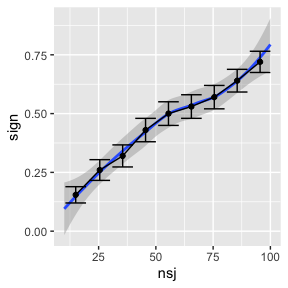# determine number of subjects needed for a power of 50% m0 <- loess(sign ~ nsj, data=COF) COF$pred <- predict(m0)
idx <- COF$pred>0.5 min(COF$nsj[idx]) # 84
##  56

# 10 Logistic GLMM

We can also simulate data from a logistic GLMM. This can be implemented by setting the argument family="binomial". Note, however, that the argument empirical=TRUE does not work for family="binomial". To be precise, the random effects for subjects or items can still be sampled using empirical=TRUE; however, the residual Bernoulli noise is not simulated exactly.

design <-
fixed.factor("X", levels=c("X1", "X2")) +
random.factor("Subj", instances=50) +
random.factor("Item", groups="X", instances=10)
dat <- dplyr::arrange(design.codes(design), Subj, Item)[c(3, 1, 2)]
(contrasts(dat$X) <- c(-1, +1)) ##  -1 1 dat$Xn <- model.matrix(~X,dat)[,2]

fix      <- c(0.5,2) # specify fixed-effects
sd_Subj  <- c(3  ,1) # specify subject random effects (standard deviation)
sd_Item  <- c(1)     # specify item random effects (standard deviation)
dat\$ysim <- simLMM(form=~ X + (X | Subj) + (1 | Item), data=dat,
Fixef=fix, VC_sd=list(sd_Subj, sd_Item), CP=0.3,
empirical=FALSE, family="binomial")
## Data simulation from a logistic generalized linear mixed-effects model (GLMM)
## Formula: binomial ~ 1 + X1 + ( 1 + X1 | Subj ) + ( 1 | Item )
## empirical = FALSE
## Random effects:
##  Groups    Name          Std.Dev.Corr
##  Subj      (Intercept)   3.0
##            X1            1.0     0.30
##  Item      (Intercept)   1.0
## Number of obs: 1000, groups:  Subj, 50; Item, 20
## Fixed effects:
## (Intercept)          X1
##         0.5         2.0
dat %>% group_by(X) %>% summarize(M=mean(ysim))
## # A tibble: 2 × 2
##   X         M
##   <fct> <dbl>
## 1 X1    0.322
## 2 X2    0.788
glmer(ysim ~ X + (X | Subj) + (1 | Item), data=dat, family="binomial", control=lmerControl(calc.derivs=FALSE))
## Warning in glmer(ysim ~ X + (X | Subj) + (1 | Item), data = dat, family =
## "binomial", : please use glmerControl() instead of lmerControl()
## Generalized linear mixed model fit by maximum likelihood (Laplace
##   Approximation) [glmerMod]
##  Family: binomial  ( logit )
## Formula: ysim ~ X + (X | Subj) + (1 | Item)
##    Data: dat
##       AIC       BIC    logLik  deviance  df.resid
##  696.9263  726.3729 -342.4632  684.9263       994
## Random effects:
##  Groups Name        Std.Dev. Corr
##  Subj   (Intercept) 3.631
##         X1          1.251    0.23
##  Item   (Intercept) 1.450
## Number of obs: 1000, groups:  Subj, 50; Item, 20
## Fixed Effects:
## (Intercept)           X1
##      0.8765       2.8495

We don’t execute the power simulation here, since this would take a long time. But it should be a straightforward extension from the other analyses shown in detail.